Q-Chem 5.1 User’s Manual

# 5.9 Double-Hybrid Density Functional Theory

Double-hybrid density functional theory (DH-DFT) has demonstrated tremendous potential for approaching the chemical accuracy with a computational cost comparable to the second-order Møller-Plesset perturbation theory (MP2). In a DH-DFT, a Kohn-Sham (KS) DFT calculation is performed first, followed by a treatment of non-local orbital correlation energy at the level of second-order Møller-Plesset perturbation theory (MP2). This MP2 correlation correction includes a a same-spin (ss) component,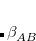, as well as an opposite-spin (os) component,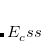, which are added to the total energy obtained from the KS-DFT calculation. Two scaling parameters,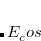and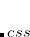, are introduced in order to avoid double-counting correlation: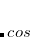(5.60)

A starting point for understanding where a functional form like Eq. eq:dft2a might come from is the adiabatic connection formula that provides a rigorous way to define double-hybrid functionals. One considers an adiabatic path between the fictitious non-interacting KS reference system (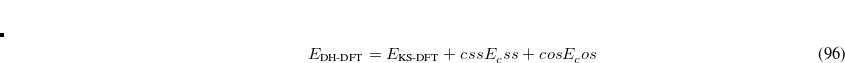) and the real physical system (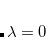), while holding the electron density fixed at its value for the real system, for all. Then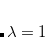(5.61)

whereis the exchange-correlation energy at a coupling strength, meaning that the exchange-correlation energy if the electron–electron terms in the Hamiltonian had the form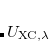rather than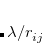. Using a linear model of this quantity,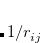(5.62)

one obtains the popular hybrid functional that includes the HF exchange (or occupied orbitals) such as B3LYP. If one further uses the Gorling-Levy’s perturbation theory (GL2) to define the initial slope at= 0, one obtains the doubly hybrid functional form in Eq. eq:dft2a that includes MP2 type perturbative terms (PT2) involving virtual orbitals: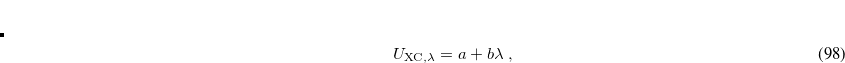(5.63)

The adiabatic connection formula has been used to develop double hybrid functionals such as XYG3. Note that XYG3 as implemented in Q-Chem uses B3LYP orbitals to generate the density and evaluate the PT2 terms. This is different from B2PLYP, an earlier doubly hybrid functional from Grimme. The latter uses truncated KS orbitals while XYG3 uses converged KS orbitals to evaluate the PT2 terms. The performance of XYG3 is not only comparable to that of the G2 or G3 theory for thermochemistry, but barrier heights and non-covalent interactions, including stacking interactions, are also very well described by XYG3.

Note:  The recommended basis set for XYG3 is 6-311+G(3df,2p).

Due to the inclusion of PT2 terms, the cost of double-hybrid calculations is formally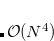, as in conventional MP2, thereby not applicable to large systems and partly losing DFT’s cost advantages. However, the highly successful SOS-MP2 and local SOS-MP2 algorithms in Q-Chem can be leveraged to develop double-hybrid functionals based on these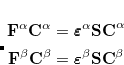methods. A version of XYG3 that uses SOS-MP2 is the XYGJ-OS functional. This functional has 4 parameters that are optimized using thermochemical data. It is not only faster than XYG3, but comparable to XYG3 (or perhaps slightly better) in accuracy. If the local SOS-MP2 algorithm is applied, the scaling of XYGJ-OS is further reduced to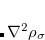. Recently, XYGJ-OS became the first double-hybrid functional whose analytic energy gradient has been derived and implemented.

Other more empirical double-hybrid functionals have been implemented in Q-Chem. Among the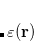B97 series of functionals,B97X-2 is a long-range corrected double hybrid that can greatly reduce the self-interaction errors (due to its high fraction of HF exchange), and has been shown significantly superior to other functionals for systems with both bonded and non-bonded interactions. Due to the sensitivity of PT2 correlation energy with respect to the choices of basis sets,B97X-2 was parameterized with two different basis sets: theB97X-2(LP) was parameterized for use with 6-311++G(3df,3pd), whileB97X-2(TQZ) was parameterized with the T/Q (triple-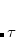/quadruple-) extrapolation to the basis set limit. A careful reading of Ref. Chai:2009 is highly advised before using either of these two functionals.

Job control variables for double-hybrid DFT are described below. Note that the PT2 correlation energy can also be computed with the efficient resolution-of-identity (RI) methods; see Section 6.6.

DH
 Controls the application of DH-DFT scheme.

TYPE:
 LOGICAL

DEFAULT:
 FALSE

OPTIONS:
 FALSE (or 0) Do not apply the DH-DFT scheme TRUE (or 1) Apply DH-DFT scheme

RECOMMENDATION:
 NONE

The following to $rem variables pertain to theB97X-2(LP) andB97X-2(TQZ) functionals. SSS_FACTOR  Controls the strength of the same-spin component of PT2 correlation energy. TYPE:  INTEGER DEFAULT:  0 OPTIONS: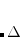Corresponding toin Eq. (5.60). RECOMMENDATION:  NONE SOS_FACTOR  Controls the strength of the opposite-spin component of PT2 correlation energy. TYPE:  INTEGER DEFAULT:  0 OPTIONS:Corresponding to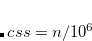in Eq. (5.60). RECOMMENDATION:  NONE Example 5.55 Applications of B2PLYP functional to LiH. $comment
Single-point calculation on LiH without RI, followed by a single-point
calculation with RI.
$end$molecule
0 1
H
Li H 1.6
$end$rem
JOBTYPE     sp
EXCHANGE    B2PLYP
BASIS       cc-pvtz
$end @@@$molecule
$end$rem
JOBTYPE     sp
EXCHANGE    B2PLYP
BASIS       cc-pvtz
AUX_BASIS   rimp2-cc-pvtz
$end  Example 5.56 Application ofB97X-2(TQZ) functional (with and without RI) to LiH molecules. $comment
Single-point calculation on LiH (with RI).
$end$molecule
0 1
H
Li H 1.6
$end$rem
JOBTYPE     sp
EXCHANGE    omegaB97X-2(TQZ)
BASIS       cc-pvqz
AUX_BASIS   rimp2-cc-pvqz
$end @@@$comment
Single-point calculation on LiH (without RI).
$end$molecule
$end$rem
JOBTYPE      sp
EXCHANGE      omegaB97X-2(TQZ)
BASIS      cc-pvqz
$end  In the following example of XYG3, Q-Chem automatically performs a B3LYP calculation first, then uses the resulting orbitals to evaluate the PT2 correlation terms. Once can also use XYG3 combined with the RI approximation; use EXCHANGE = XYG3RI to do so, along with an appropriate choice of auxiliary basis set. Example 5.57 XYG3 calculation of N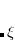$molecule
0 1
N      0.000000   0.000000   0.547775
N      0.000000   0.000000  -0.547775
$end$rem
EXCHANGE  xyg3
BASIS     6-311+G(3df,2p)
$end  The next example illustrates XYGJ-OS. This functional uses the RI approximation by default, so it is necessary to specify an auxiliary basis set. Example 5.58 XYGJ-OS calculation of N$molecule
0 1
N      0.000000   0.000000   0.547775
N      0.000000   0.000000  -0.547775
$end$rem
EXCHANGE    xygjos
BASIS       6-311+G(3df,2p)
AUX_BASIS   rimp2-cc-pVtZ
PURECART    1111
TIME_MP2    true
$end  The final example uses the local version of XYGJ-OS, which is the same as the original XYGJ-OS but with the use of the attenuated Coulomb metric to solve the RI coefficients. Here, the keyword omega determines the locality of the metric. Example 5.59 Local XYGJ-OS calculation of N$molecule
0 1
N    0.000    0.000   0.54777500
N    0.000    0.000  -0.54777500
$end$rem
EXCHANGE   lxygjos
OMEGA      200
BASIS      6-311+G(3df,2p)
AUX_BASIS  rimp2-cc-pVtZ
PURECART   1111
\$end